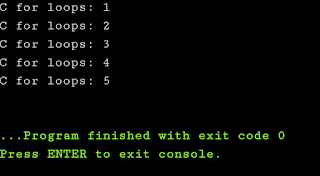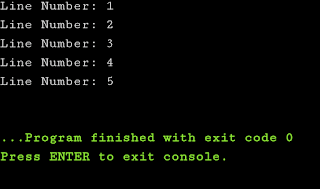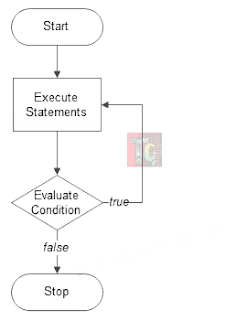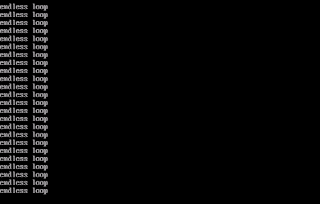# For, While, Do-While Loops in C

In certain programs, we come across a situation where there needs to iterations with a certain set of statements. Such statements if written repeatedly will consume a lot of time and here is where looping statements jump in. In this article, we'll see different types of loops in C (for, while, do-while and infinite), their uses and sample programs. Let's get started.Loops in C

## What are Loops in C?

To understand loops in C programming we can take a look at the following example.

Imagine your teacher asked you to write 15 times "I'll do my homework" by writing a C Program. (Usually, that wouldn't be the case unless it's computer practical class)

Now you have two approaches for writing that. One by one, we'll see how.

### Iterative Method

Under this method you can type the printf() statement 15 times and show the required output.

``````#include<stdio.h>
int main()
{
printf("I'll do my homework\n");
printf("I'll do my homework\n");
printf("I'll do my homework\n");
printf("I'll do my homework\n");
printf("I'll do my homework\n");
printf("I'll do my homework\n");
printf("I'll do my homework\n");
printf("I'll do my homework\n");
printf("I'll do my homework\n");
printf("I'll do my homework\n");
printf("I'll do my homework\n");
printf("I'll do my homework\n");
printf("I'll do my homework\n");
printf("I'll do my homework\n");
printf("I'll do my homework\n");
printf("I'll do my homework\n");
return 0;
}``````

#### Output:Output of Iterative Approach

### Using Loops

In this method, you'll have to type the output statement once but enclose it within a loop. Here's how:

``````#include<stdio.h>
int main()
{
int i,n=15;
for(i=1;i<=n;i++)
{
printf("I'll do my homework\n");
}
return 0;
}``````

#### Output:Output of Iterative Approach

We can understand that a loop is a set of commands repeated until a prescribed condition is met.
• An operation is carried out, such as input of data and changing it, and then some condition is checked, i.e., if a counter has reached a prescribed value.
• If the counter has not reached the desired value, the next command in the loop returns to the first command in the loop and repeat it.
• If the counter has been reached, the next loop jumps to the successive command or quits the loop.

## Types of Loops in C:

Based on the flow of control there are two types of loops:
• Entry controlled loop: Here the test condition is tested before entering the loop body. For Loop and While Loop fall under this category.
• Exit controlled loop: However, here the test condition is evaluated at the end of the loop body. Thus, the loop body will execute at least once, irrespective of whether the test condition is true or false. do – while loop falls under this category.
We'll now get a clear idea about different looping statements in C.

## Nature of for loop in C

As we've learned before, for loop is an entry controlled loop. Just like the basic nature of a looping statement, a for loop acts as repetition control structure. It lets us carry out a particular set of statements n number of times until the prescribed condition is met. The syntax of a for loop will make it clearer.

## Syntax of for loop

The syntax of for loop is as follows:
``````for(initialization expression;test expression;update expression)
{
// body of the loop
// statements to be executed
}``````

Clearly, from the above syntax, we can conclude at first the loop variable is initialized to some value, then a check is carried out whether this variable is less than or greater than counter value. When the statement is found true, the loop body is executed and the loop variable gets updated. Steps are repeated until the exit condition arrives.
The parts of a for loop are detailed as under:
• Initialization Expression: Here we have to initialize the loop counter to some value. Usually, the value is initiated at 0 or 1, but as per needs, those values are changed.
• Test Expression: As the name goes, here the validity of expression is tested. If the condition results to true then loop will be executed following which the expression is updated else the loop will exit.
• Update Expression: After executing loop body this expression updates (increases/decreases)  the loop variable by some prescribed value.

### Flowchart of for loop:Flowchart of for loop

A sample program using for loop in C:

``````#include<stdio.h>
int main()
{
int n,times=5;
for(n=1;n<=times;n++)
{
printf("C for loops: %d\n",n);
}
return 0;
}``````

#### Output:For loop output

## Nature of while loop in C

The while loop has a single control condition and executes the codes within it as long the condition is true. Here the condition of the loop is tested before the body of the loop is executed. This loop is useful where we are not certain about the exact number of iterations of loop beforehand.

## Syntax of while loop

The syntax of while loop is as follows:

``````initialization expression;
while (test_expression)
{
// statements update_expression;
}``````

What differs in every loop is the placement of fundamental quantities i.e., initialization expression, test expression and update expression.

### Flowchart of while loop:

A sample program using while loop in C:

``````#include<stdio.h>
int main()
{
int n=1,times=5;
while(n<=times)
{
printf("Line number: %d\n",n);
n++;
}
return 0;
}``````

#### Output:While loop output

## Nature of do-while loop in C

In do-while loop the condition is tested at the end of the loop body, i.e do while loop is exit controlled whereas the do loop is entry controlled loop. In do-while loop, the loop body will execute at least once irrespective of the test condition.

## Syntax of do-while loop

The syntax of do-while loop is as follows:

``````initialization expression;
do
{
// statements update_expression;
}while(test_expression);
``````

### Flowchart of do-while loop:Flowchart of do-while loop

A sample program using do-while loop in C:

``````#include<stdio.h>
int main()
{
int n=1,times=5;
do
{
printf("Line Number: %d\n", n);
n++;
}while(n<=times);
return 0;
}``````

#### Output:Do-while loop output

## When to use which loop?

• When the number of iterations is known beforehand use for loop.
• When the exact number of iterations is not known but the loop termination condition is not known use while loop.
• If the code needs to be executed at least once use do-while loop.

## Are there infinite loops?

If you've observed the above looping statements, all of them have a condition statement or test expression. Once the counter's value matches that the loop quits. But what if there were no exit condition?

In such case, because there is an absence of limiting criteria the loop will be executed endlessly. Such an issue is noticed in the following program:

Sample program to check infinite loop:

``````#include<stdio.h>
int main()
{
int i=1;
while(i<10)
{
printf("endless loop\n");
}
}``````

#### Output:Infinite Loop in C

All programs written in this post are compiled online.

### Books I Prefer:I hope this article was helpful to understand the types and nature of looping statements in C! Comment below, if you've got any question. Head back soon for another interesting article on C Programming.